# Who needs a calculator? Multiplying with Your Fingers

To do multiplication with your fingers in binary is very easy: it’s just a mixture of addition and bit-shifting. The only real trick is memory: to multiply a×b, you need to remember the binary digits of both x and y, which can be a bit of a trick for 10 digit binary numbers.
The trick that I like is to use coins. Lay out a bunch of coins: one for each binary digit of a, and one for each binary digit of b. Put two lines on a piece of paper: one line will be the ones, the other will be the zeros. So, for example, to multiple 47 times 24, you’d start with the following: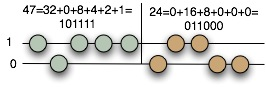The coins on the paper are your guide, to help you remember the two numbers you’re multiplying. Now the basic algorithm. You start with zero on your fingers; then, starting with the *largest* digit of *B*:
1. Take the current sum on your hands, and multiply it by two. Multiplying by
two is just a simple shift operation – shift each digit up to the next highest
position.
2. If the current digit is “1”, then add “A” to what’s on your fingers.
3. Move to the next digit of “B”.
So, for example: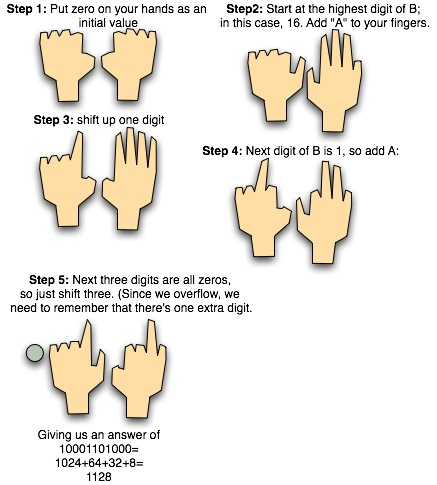That’s all there is to it. It’s really easy; once you’ve
to multiplication this way is very straightforward and
mechanical.
For the particularly clever folks out there, you’ll notice that this is
pretty much the same algorithm that we used for [multiplying roman numerals](http://scienceblogs.com/goodmath/2006/08/roman_numerals_and_arithmetic.php).

# Binary Fingermath

There is another way of doing math on your fingers, which gives you a much greater range of numbers, and which makes multiplication particularly easy. It’s a bit more work to get used to than the finger abacus, but it has a lot less limitations. Someone in the comments of the finger-abacus post mentioned that they do something similar.
The methods for binary fingermath that I’ll describe are my own creation; so if you think they’re ridiculous, the blame is entirely mine. I know other people have come up with similar things, but this is my own personal variant.

# No Abacus Handy? Use your hands.

Suppose you want to do some math, but you don’t have an abacus handy. Oh, the horror! What do you do?
No problem! Your hands make a *great* two-digit soroban-type abacus. The four beads on the lower deck are your four fingers; the bead on the upper deck is your thumb, as illustrated in this diagram (with apologies for my terrible artwork):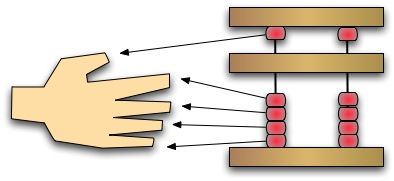So the numbers from one to nine look like:To get two digits, you use your right hand for the ones, and your left for the tens. So, for example, let’s look at a simple addition: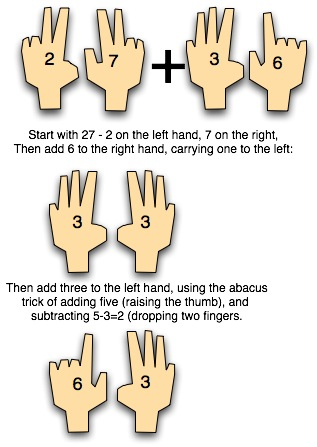Once you know the abacus, doing this with your hands is pretty simple. It’s definitely a limited technique, since you can’t get past one hundred without using your toes, but it’s a nifty trick, and it’s easy to teach kids to do this for working out math problems. If you’ve got a kid who’s a tactile thinker, it’s amazing how much learning to do this can help them. I’ve seen kids who do paper math with many digits by working out subparts of the problem using this style of finger-abacus.
There’s actually a whole Korean teaching method for math called something like chisan-bop. From what I understand, they build up on this quite a bit, to be able to do much more complicated stuff than just two-digit addition, but I haven’t been able to find an english textbook on chisan-bop. All the english texts basically show what I just did above: the two-digit abacus on the fingers.

# Square Root on the Abacus

Doing square root on the abacus is a lot like doing it on paper. The big difference? It’s actually *easier* on the abacus. What I find pretty cool is that I’m a rank beginner at the abacus. I never actually tried to use one before I started writing these posts. But I can do that root *faster* on the abacus than I can on paper.
The one difficult step in the paper square root is guessing the approximate digits; as you get beyond the third or fourth digit, the numbers start getting a bit large, and it can be hard to guess the correct estimate. On the abacus, you can very rapidly do repeated subtraction, so you deliberately guess low, and then add on. You’ll see what I mean as we work through an example.
One thing about the square root is you need a bigger abacus. So far, we’ve used very small ones for the examples here. The more you want to do with an abacus, the bigger you want it to be. A small abacus typically has something like 9 columns; a medium abacus has 13 digits. But for more interesting calculations, the kind of thing that we westerners would have a slide rule or scientific calculator, the abacus equivalent is a *27* digit abacus with a couple of sliding markers for helping keep track of things. You want a nice big abacus for doing things like roots, because you’re going to partition it into multiple sections.

# Computing Square Roots on Paper

To do a square root on an abacus, you use partitions to do a paper algorithm for square root using the abacus. The catch is that most people don’t even *remember* how to do square roots on paper, if they ever learned it at all. (In fact, in school, *I* didn’t learn the classical paper algorithm; we never really did roots on paper; the closest we did was using square root as an example of Newton’s method. Like so much of my basic math, I learned this from my father.)
So, for your entertainment and edification, today, I’ll describe the classical algorithm for computing square roots on paper. I’ll also show you just why it works.

# Division on the Abacus

Now we’re going to try something challenging on the abacus: *division*. Like multiplication, abacus division is close to the way you’d do it on paper. But just like doing paper division is trickier than paper multiplication, abacus division is tricker than abacus multiplication. But the technique that is used to do division on the abacus is an important fundamental one: it’s what makes it possible to use the abacus for more advanced operations, like roots.

# Using the Abacus, part 2: Multiplication

Once you can add on an abacus, the next thing to learn is multiplication. Like addition, it follows pretty closely on the old pencil-and-paper method. But it’s worth taking the time to look closely and see it step by step, because it’s an important subroutine (to use a programming term) that will be useful in more complicated stuff.

# Arithmetic on the Abacus: Part 1

If you want to talk about mechanical computing tools, you can’t ignore the abacus. It’s the oldest computing tool in the world; and it’s still very commonly used. It’s also about as different from the slide rule as you could imagine. The abacus is really fundamentally an addition device; the slide-rule is fundamentally a multiplier. And the slide rule is very complicated – all those different scales, in logarithmic relationships; the abacus is thoroughly simple – just beads hanging on wires. But don’t let that fool you: the abacus is is a remarkable device, which is capable of a really huge number of computations: addition. subtraction, multiplication, division, even square and cube roots.
The abacus is, basically, sort of like a *better* piece of paper. Any kind of numerical calculation that you can do using piece of paper and a pencil, you can do on an abacus; only it’s a whole lot faster on the abacus.

# Using a Slide Rule Part 2: Exponents and Roots

Slides rules are actually astonishingly powerful things. The simple slide rule does multiplication and division using the C and D scales; strictly speaking, you can have a basic rule with nothing but C and D. But you almost never see a rule that simple. (The only one I’ve ever seen with only the two scales was a circular rule used as a promotional giveaway.)

The other scales are where things get a bit complicated; but it’s a lot of fun to figure out how they work, and to see how much you can actually do with, basically, two attached rulers with a bunch of different markings.

As an example, I’m going to walk through how you do exponents and roots using a slide rule. They’re interesting, because they’re the hardest things to do on the slide rule; so if you can get how to do them, there’s pretty much nothing that you can do with a slide rule that you won’t be able to figure out by understanding the scale. I’m using the same virtual slide rule program as yesterday, but this time, the images are of a Pickett N3-T, which is a log-log duplex trig scientific rule. We’ll need the extra scales of a log-log for today.

One quick note of clarification: a slang term for a slide rule is a “slipstick”, or just “stick”. I’m actually used to calling it a stick; that’s what my father used to call it when he taught me to use it. So if I slip up and say “stick”, you’ll know what I’m talking about.

# Manual Calculation: Using a Slide Rule (part 1)

Several people in the geekout thread asked me to explain how a sliderule works, and I’ve been meaning to write a couple of article about manual computing devices. So I thought I’d do it. There’s a nice slide-rule simulator at Derek’s Virtual Slide Rule Gallery, which is what I used to generate the images in this article.

I know a lot of people think that the idea of learning to use something like a slide rule is insane in an age of computers and calculators, and that this is a silly thing to post about. But I really love slide rules, and not just because I’m a geek. Slide rules make math tactile. Using a slide rule makes you understand how certain kinds of math work; and not just a theoretical understanding, but an understanding on a very concrete, physical level. My dad taught me to use one not because I needed to know (I’m not that old!), but because he loved it and thought it was cool; my slide rule is the one that he used in college. He gave it to me when I was in high school. It’s a beautiful K&E log-log duplex decitrig.

There are a couple of things to be said about slide rules up front. They’re beautiful things, and the guy who invented them is an incredible genius. But they’re not a tool for the weak-of-heart. Using a slide rule isn’t like using an electronic calculator. You actually need to do an approximation of the calculation in your head, because the slide rule doesn’t do powers of ten; you need to do that by yourself! Also, in general, the slide rule is used for the “hard stuff”; multiplication and division, logarithms, exponents, and trigonometry. Addition and subtraction you do by yourself, either in your head, or on paper.

The basic idea of the slide rule comes from logarithms, in particular this fundamental identity: x * y = blogb(x) + logb(y). That is: adding logarithms is equivalent to multiplying numbers. The slide rule places numbers onto a ruler on a *logarithmic* scale; so the distance from “1” to a number “n” on the rule is the logarithm of “n”. That’s the whole fundamental trick to make it work.

Let’s take a look at a slide rule. This is a picture of a Pickett Microline sliderule. That’s a very simple rule, which is easy to see on the computer, but it’s relatively wimpy. It doesn’t have a lot of scales (which is equivalent to a calculator with very few buttons); and it’s really only good for 2 to 2.5 significant digits. (Personally, I’m not a pickett fan; I prefer the big old K&Es, but that’s just because they’re what I’m used to.)For multiplication and division, we only need two scales: the D scale, which is the top row of the lowest third of the rule; and the C scale, which is the bottom row of the moving slide in the center. C and D are done with the same logarithmic scale. We’ll also use the *cursor*, which is the vertical line on the transparent view slide.

Let’s say we wanted to multiply 22.5 by 3.7. We move the center slide so that “1” on the C scale lines up with 2.25 on the “D” scale below it: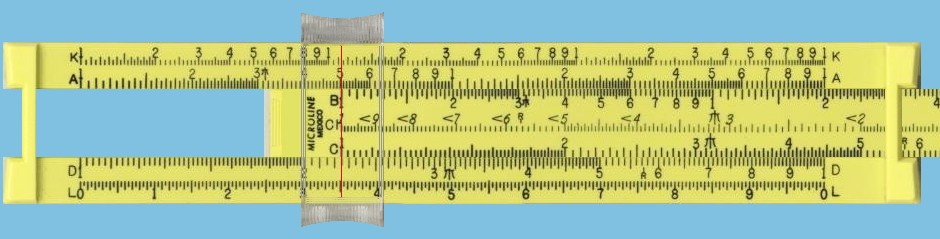Now – since adding logarithms is multiplying numbers, and the position of a number on the C and D scales are determined by the same logarithm, that means that “3.7” on the “C” scale is in the same position as “2.25*3.7” on the D scale. So what’s on the D scale at 3.7? We slide the cursor over (both to mark the position, and to make it easier to read), and find that it’s at 8.3.So the answer is 8.3 times 10 to the something. The rule doesn’t tell us what. So we do it approximately in our heads. It’s about 20 times 3 and a half, which is around 80. So the answer is 83. (The exact answer is 83.25, but this rule isn’t big enough for us to see that.)

See? Simple. Now, if we wanted to multiply that by, say, 18, we’d slide the “1” over so that it lined up with the cursor… Except that then, the answer is off the end of the rule. But no problem! There’s *also* a one on the *other* end of the rule. We can slide the C scale so that its *right hand* 1 is over 83 where we’ve left the cursor. Now we slide the cursor down to 1.8 on the C scale:And you can see it’s sitting at about 1.49. But since we only used two digits, we can only read two digits, so we say 1.5. Now we need to do our powers of ten: it’s about 20 times 80, which is 1600. So it’s 1.5×103, or about 1500. (Exact result is 1494.)

Division is almost the same thing done backwards: x/y = aloga(x) – loga(y). So, to divide x by y, we put “y” on the C scale over “x” on the D scale, and slide the cursor over to 1 on C. For example, let’s take π/2. Most rules have a specific mark for π to make that easy. So we slide 2 on C to line up with π on D: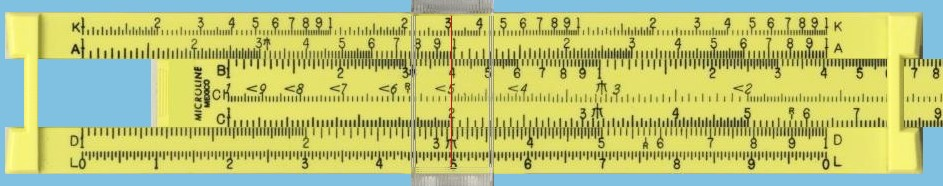And slide the cursor to one on C:And our answer is: about 1.57. (The cursor is about half-way between the marks for 1.56 and 1.58; and π is positioned to three significant digits.) We need to do the powers of ten for division to, but that’s easy; we know π/2 is between 1 and 2, so it’s 100, so the answer is just 1.57.